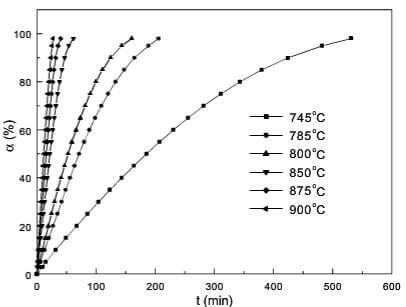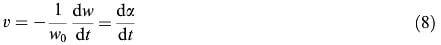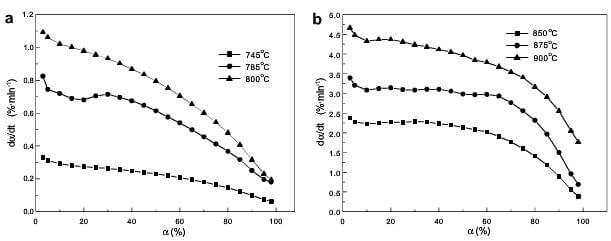# Oxidation kinetics and mechanisms of a 2D C/C composite (3)

The 2D C/C composite used in this work is a commercial product named CFC-21. The bulk density is 1.7g/cm3, the bending strength is 206Mpa and the tensile is 268Mpa. These samples for investigation have been cut of homogeneous blocks with dimensions about 4-4-2mm.

Thermogravimetric tests of 2D C/C composite were carried out by a STA449C simultaneous thermal analyzer under isothermal conditions. The samples were heated to the desired temperature for the isothermal oxidation in flowing nitrogen. When the desired temperature was reached, the sample was held at the temperature for about 5 min and then the gas was switched from nitrogen to air. All the specimens were placed in alumina pans and oxidized in a flowing air. The weight loss during oxidation was monitored as a function of time.

The microstructure of the samples before and after oxidation was observed by scanning electron microscope.

The weight loss as a function of oxidation time at different temperatures for 2D C/C composite is shown in fig.1 with increasingfig.1-Plot of α vs. t for 2D CC composite

temperature, it is obvious that the weight loss increases at identical time and the entire oxidation time decreases. It is also seen that the weight loss is a linear function of time at 0-60% and obviously non-linear over 60%.

The oxidation rate of the sample, v, can be expressed as bellow:Where dw/dt is the weight variation rate, w0 is the initial weight of the sample, and dα/dt is the differential of weight loss on time. Fig.2(a) and (b) show oxidation rate as a function of weight loss for 2D C/C composite for the various temperatures, respectively. At 745, 785 and 800°C, the oxidation rate decreases sharply in the range 0-10% and 40-100% weight loss, while a relatively slow decrease are found in the range 10-40% weight loss. At 850, 875 and 900°C, the oxidation rate decreases sharply in the range 0-10% and 60-100% weight loss, and is in stable state in the range 10-60% weight loss.fig.2-plot of dα-dt vs a for 2D CC composite